Most Affordable JEE | NEET | 8,9,10 Preparation by Kota's Top IITian Doctor Faculties

# NCERT Solutions for Class 9 Science chapter 10 - Gravitation - eSaral

Class 9Hey, are you a class 9 student and looking for ways to download NCERT Solutions for Class 9 Science Chapter 10 "Gravitation"? If yes. Then you are at the right place.

In this article, we have listed NCERT solutions for class 9 Science Physics Chapter 10 in PDF that is prepared by Kota’s top IITian’s Faculties by keeping Simplicity in mind.

If you want to learn and understand class 9 Science chapter 10 "Gravitation" in an easy way then you can use these solutions PDF.

NCERT Solutions helps students to Practice important concepts of subjects easily. Class 9 Science solutions provide detailed explanations of all the NCERT exercise questions that students can use to clear their doubts instantly.

If you want to score high in your class 9 Science Exam then it is very important for you to have a good knowledge of all the important topics, so to learn and practice those topics you can use eSaral NCERT Solutions.

So, without wasting more time Let’s start.

### Download The PDF of NCERT Solutions for Class 9 Science Chapter 10 "Gravitation"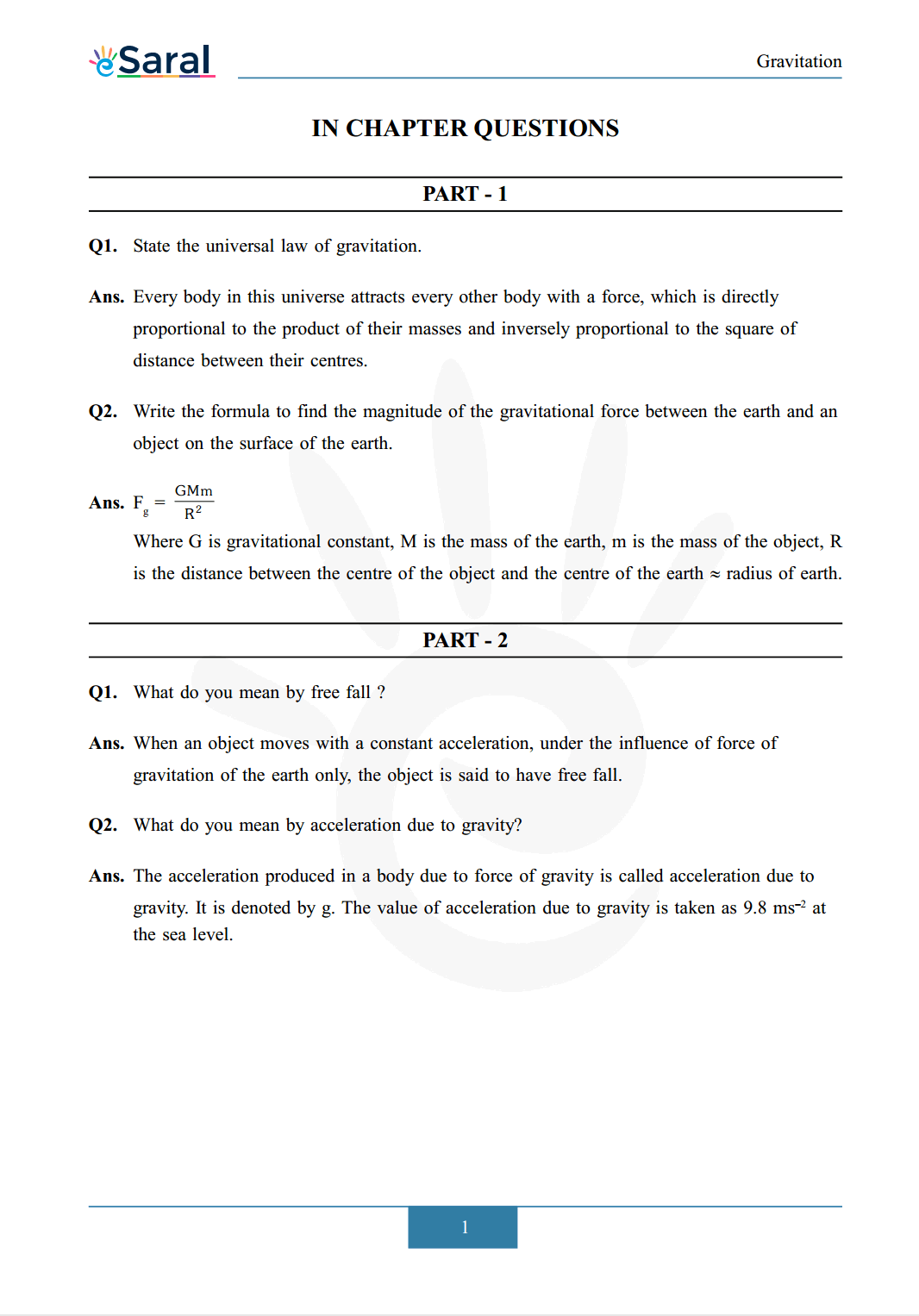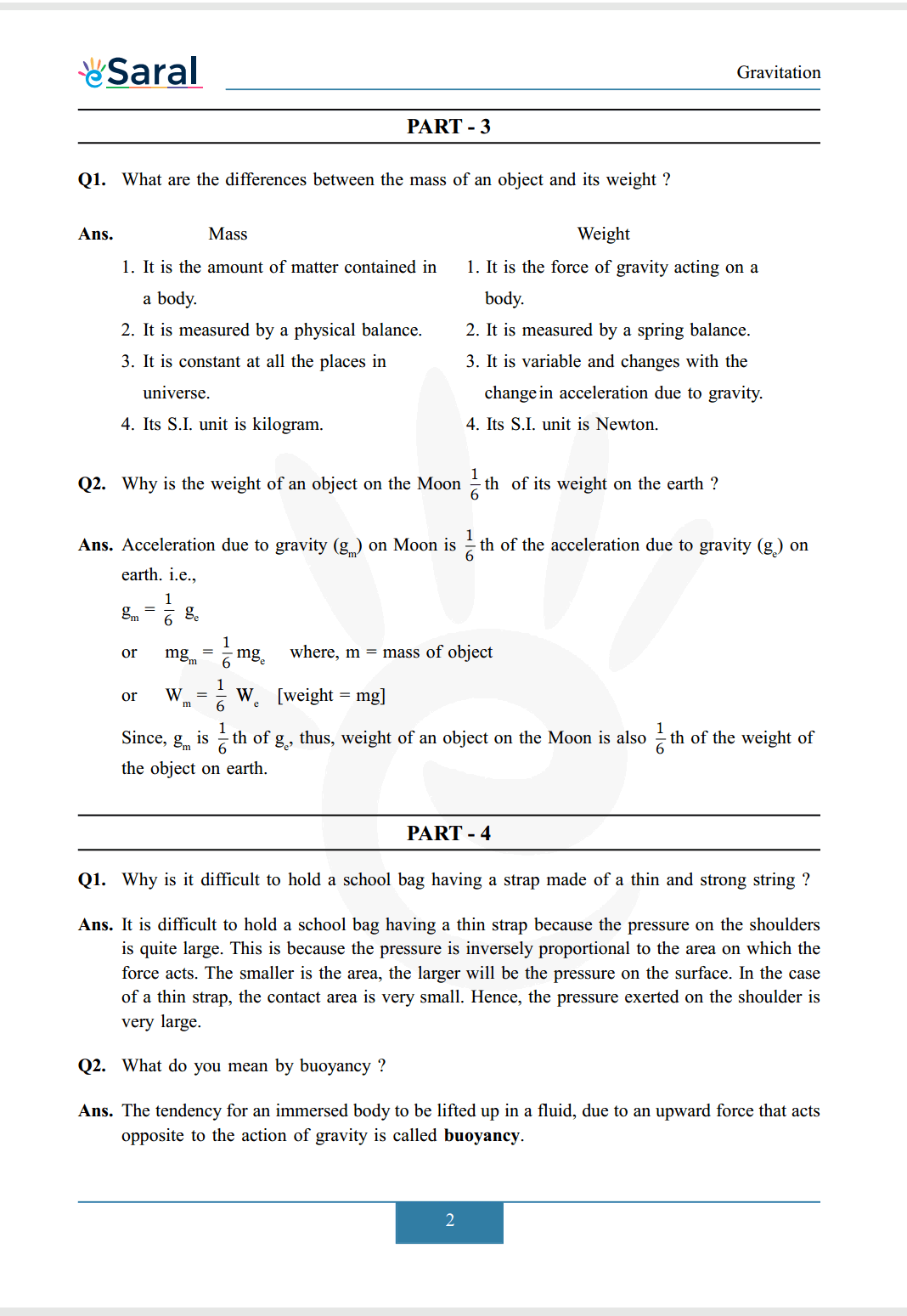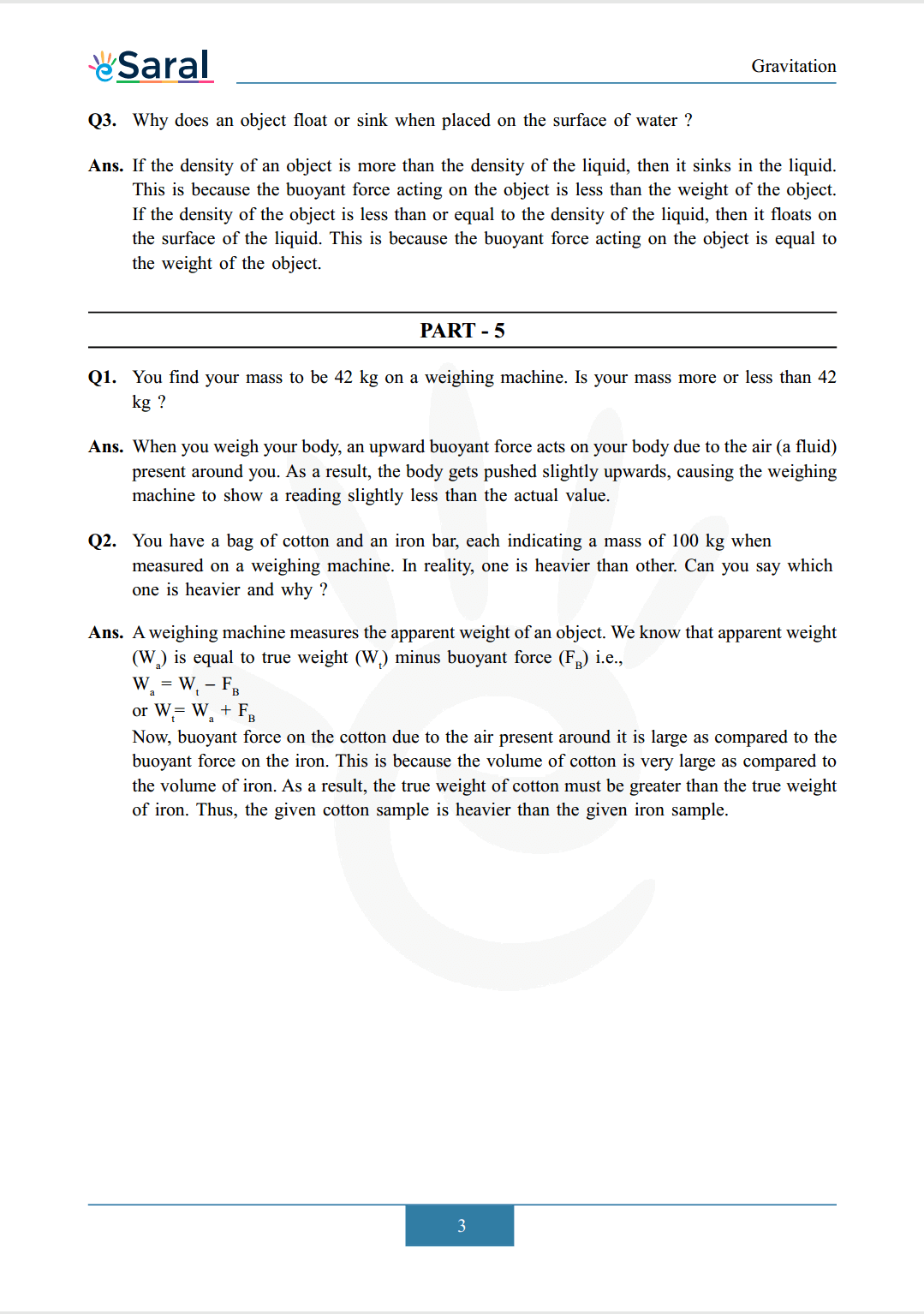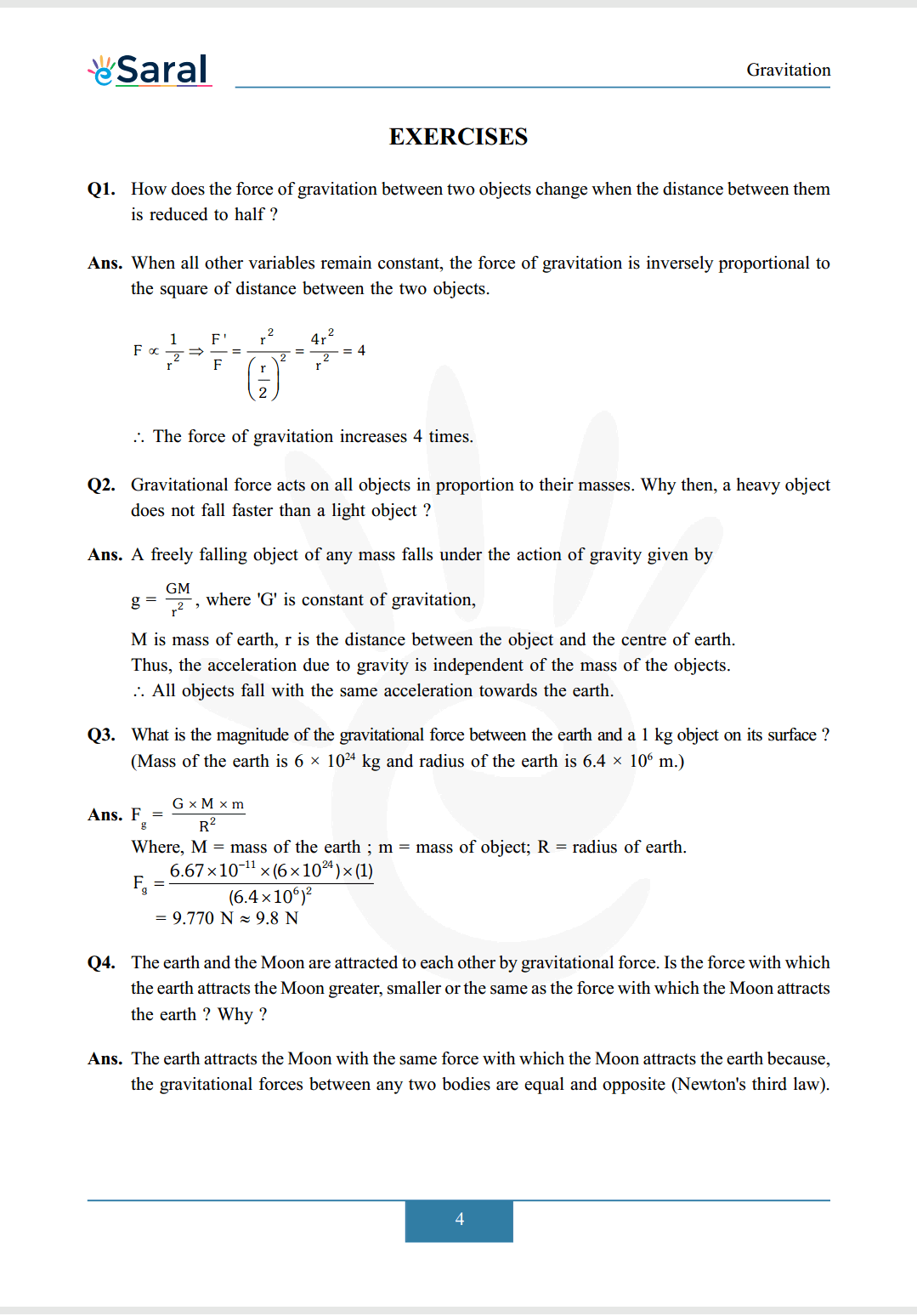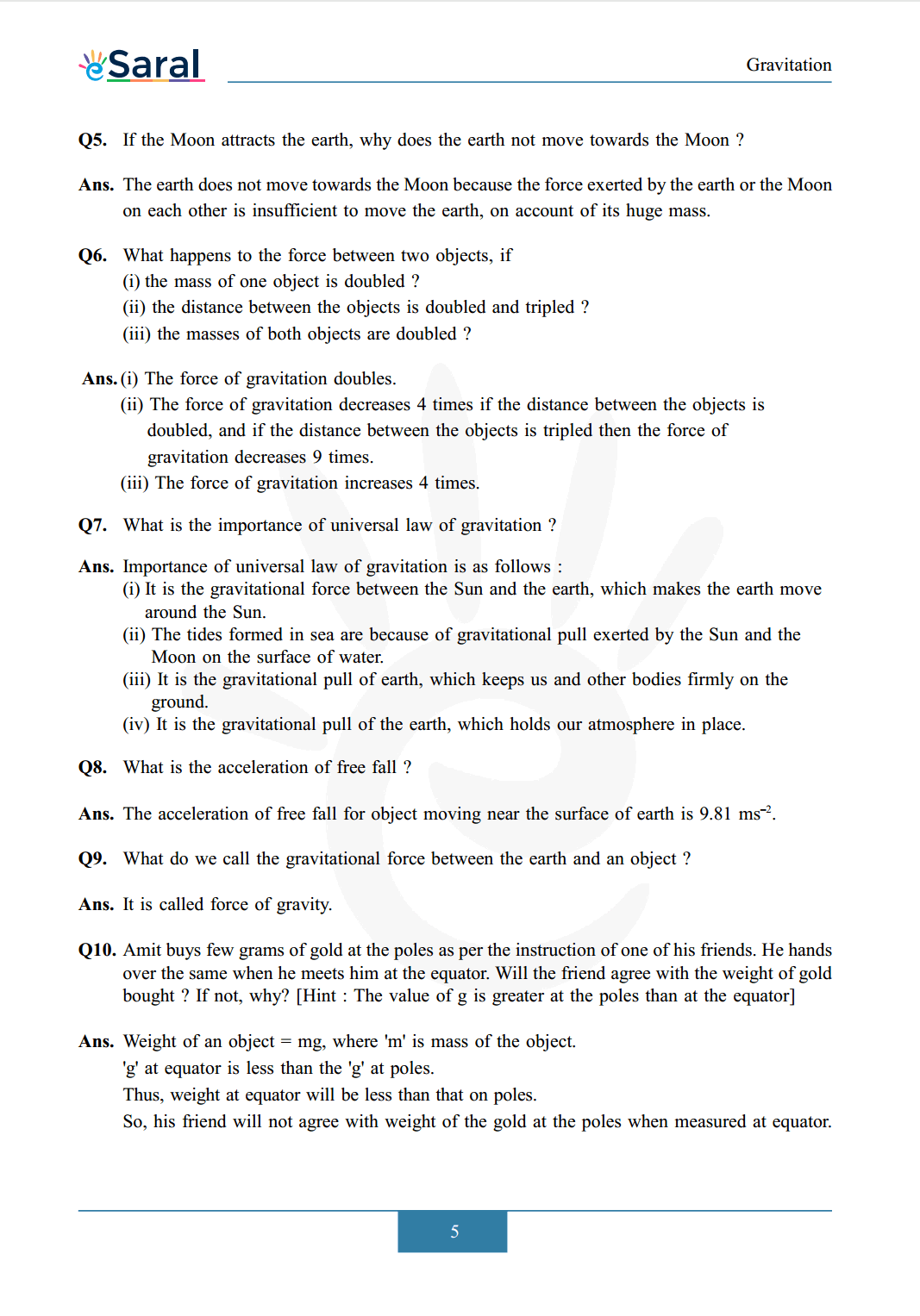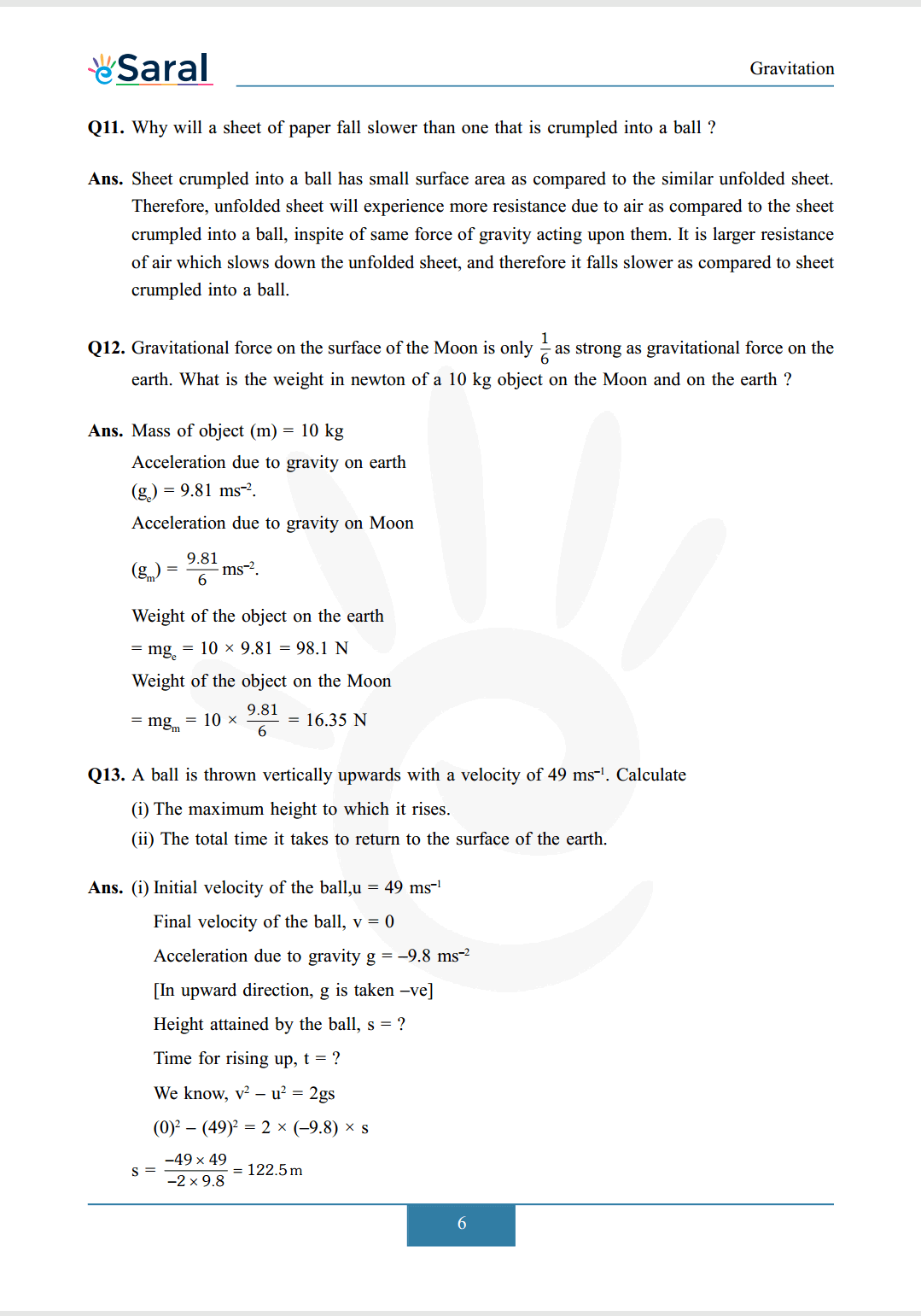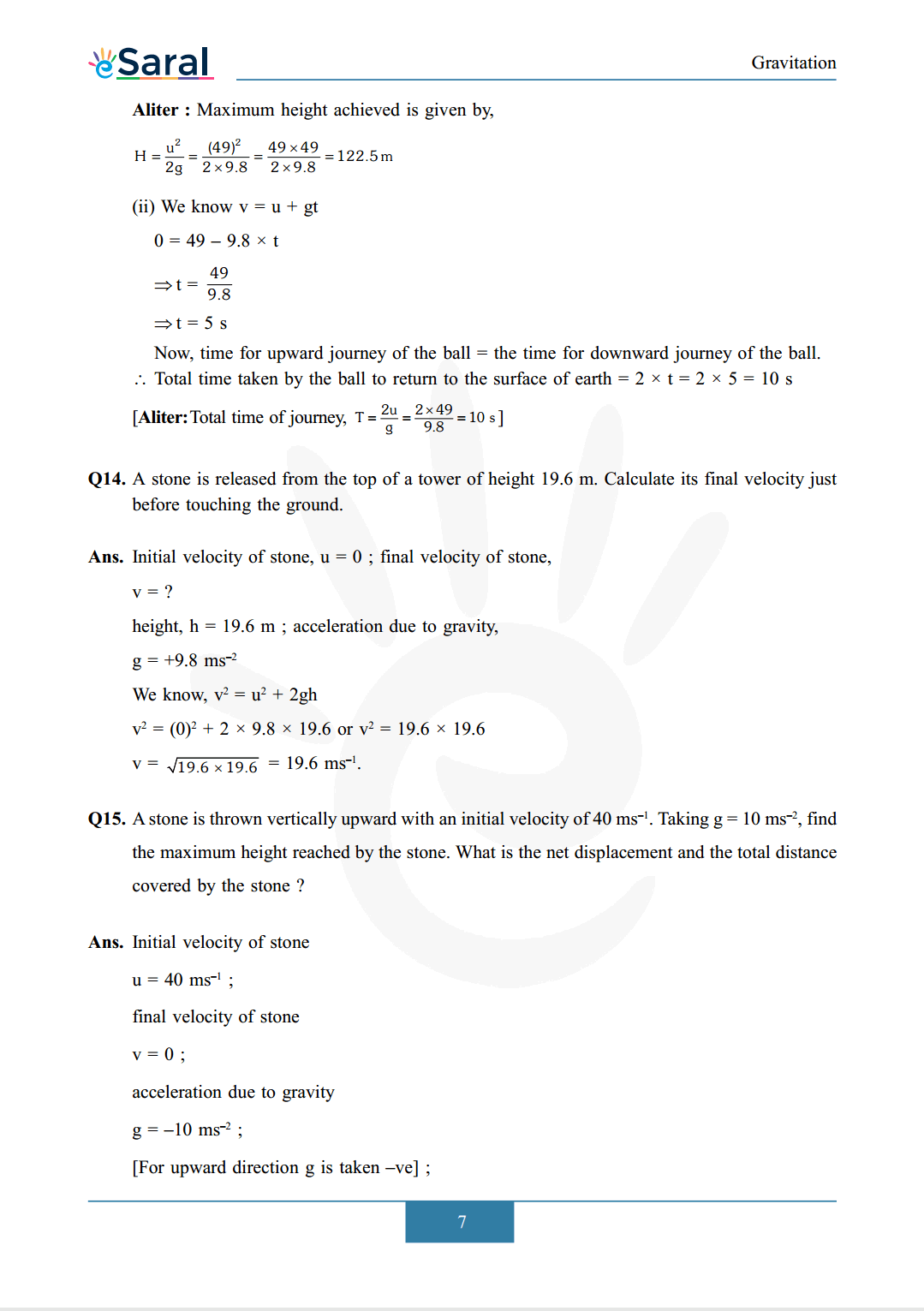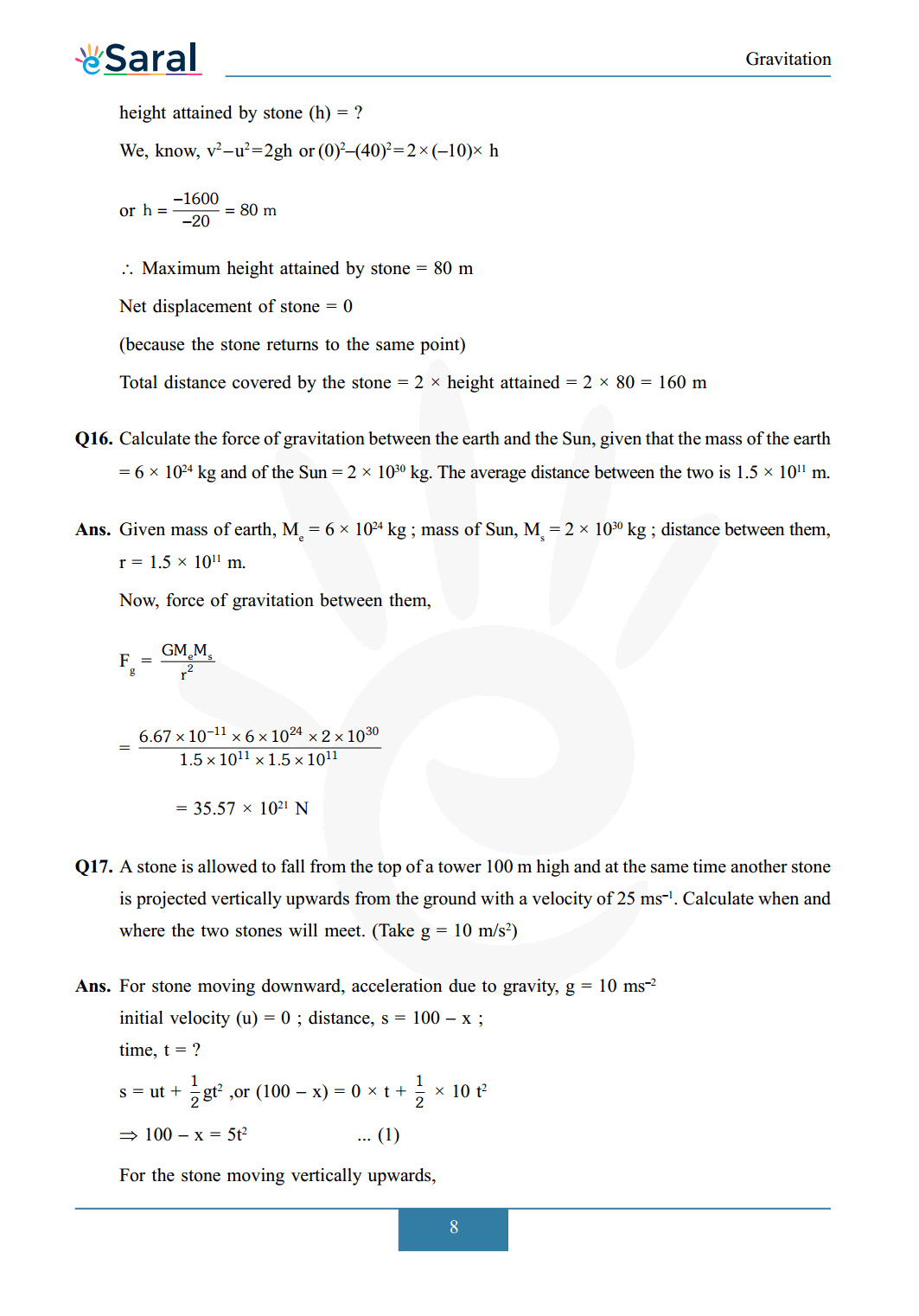###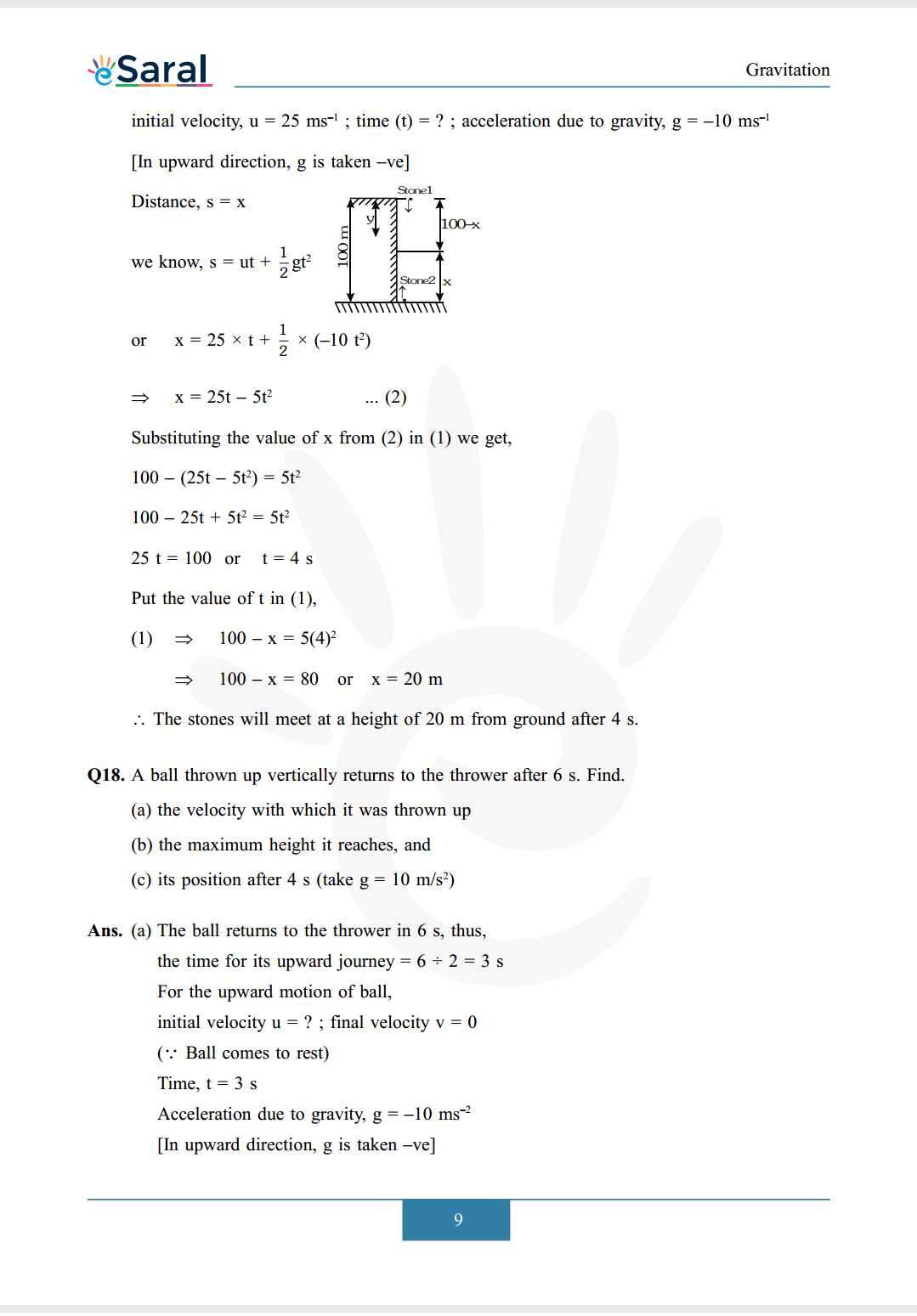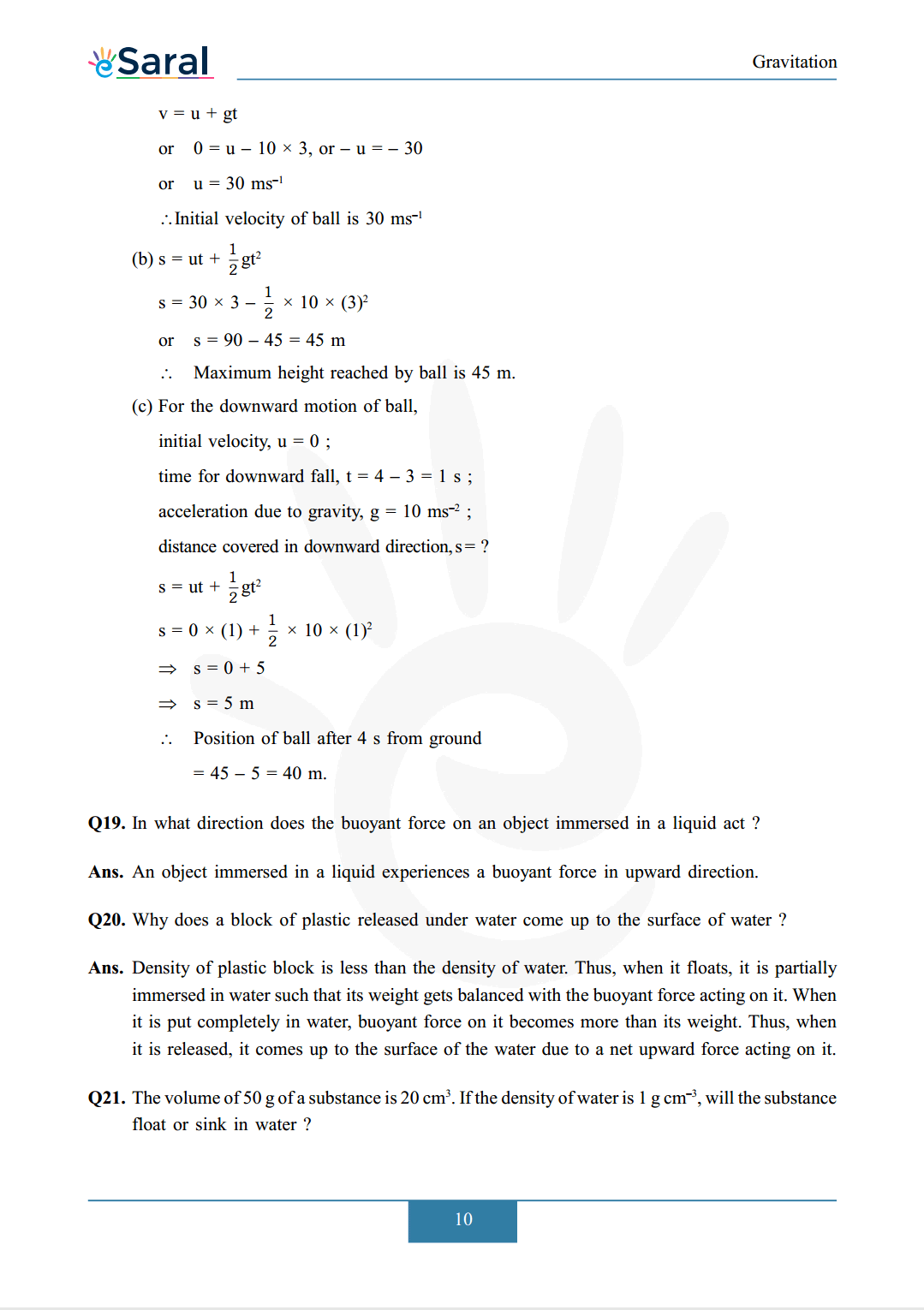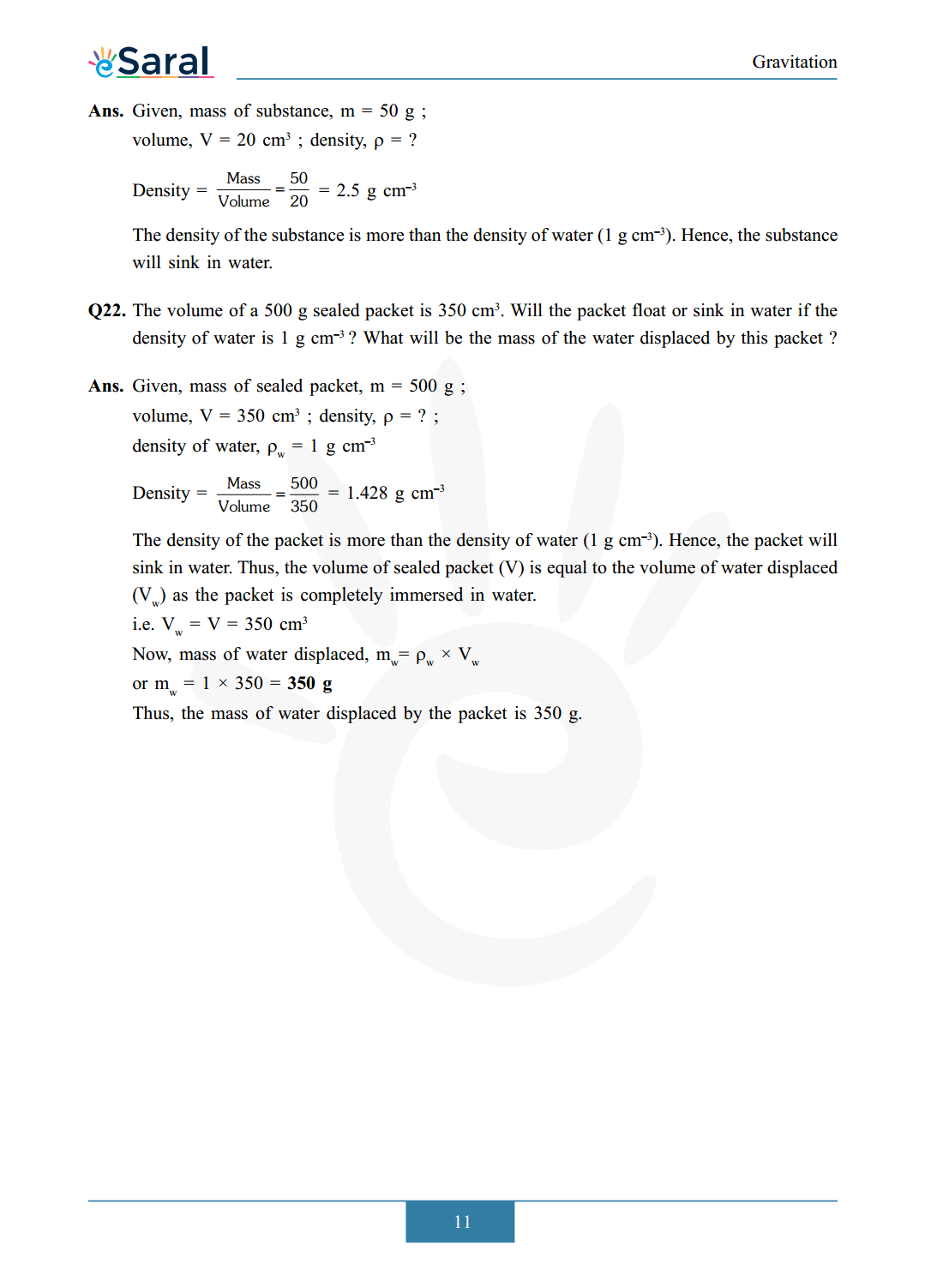Download The PDF of NCERT Solutions for Class 9 Science Chapter 10 "Gravitation"

### Important topics of class 9 Science Chapter 10

• Gravitation
• Mass
• Weight
• Free Fall
• Thrust and Pressure
• Relative Density
• Archimedes Principal

#### All Questions of Chapter 10

Once you complete the chapter 10 then you can revise it by solving following questions.

Q1.State the universal law of gravitation.

Q2.Write the formula to find the magnitude of the gravitational force between the earth and an object on the surface of the earth.

##### Part 2

Q1.What do you mean by free fall?

Q2.What do you mean by acceleration due to gravity?

##### Part 3

Q1.What are the differences between the mass of an object and its weight?

Q2.Why is the weight of an object on the Moon $\frac{1}{6}$ th of its weight on the earth ?

##### Part 4

Q1.Why is it difficult to hold a school bag having a strap made of a thin and strong string?

Q2.What do you mean by buoyancy?

Q3.Why does an object float or sink when placed on the surface of water?

##### Part 5

Q1.You find your mass to be $42 \mathrm{~kg}$ on a weighing machine. Is your mass more or less than 42 $\mathrm{kg}$?

Q2.You have a bag of cotton and an iron bar, each indicating a mass of $100 \mathrm{~kg}$ when measured on a weighing machine. In reality, one is heavier than other. Can you say which one is heavier and why?

##### EXERCISES

Q1. How does the force of gravitation between two objects change when the distance between them is reduced to half?

Q2. Gravitational force acts on all objects in proportion to their masses. Why then, a heavy object does not fall faster than a light object?

Q3. What is the magnitude of the gravitational force between the earth and a $1 \mathrm{~kg}$ object on its surface? (Mass of the earth is $6 \times 10^{24} \mathrm{~kg}$ and radius of the earth is $6.4 \times 10^{6} \mathrm{~m}$.)

Q4. The earth and the Moon are attracted to each other by gravitational force. Is the force with which the earth attracts the Moon greater, smaller or the same as the force with which the Moon attracts the earth? Why?

Q5. If the Moon attracts the earth, why does the earth not move towards the Moon?

Q6. What happens to the force between two objects, if?
(i) the mass of one object is doubled?
(ii) the distance between the objects is doubled and tripled?
(iii) the masses of both objects are doubled?

Q7. What is the importance of universal law of gravitation?

Q8. What is the acceleration of free fall?

Q9. What do we call the gravitational force between the earth and an object?

Q10. Amit buys few grams of gold at the poles as per the instruction of one of his friends. He hands over the same when he meets him at the equator. Will the friend agree with the weight of gold bought? If not, why? [Hint: The value of $\mathrm{g}$ is greater at the poles than at the equator]

Q11. Why will a sheet of paper fall slower than one that is crumpled into a ball?

Q12. Gravitational force on the surface of the Moon is only $\frac{1}{6}$ as strong as gravitational force on the earth. What is the weight in newton of a $10 \mathrm{~kg}$ object on the Moon and on the earth ?

Q13. A ball is thrown vertically upwards with a velocity of $49 \mathrm{~ms}^{-1}$. Calculate
(i) The maximum height to which it rises.
(ii) The total time it takes to return to the surface of the earth.

Q14. A stone is released from the top of a tower of height $19.6 \mathrm{~m}$. Calculate its final velocity just before touching the ground.

Q15. A stone is thrown vertically upward with an initial velocity of $40 \mathrm{~ms}^{-1}$. Taking $\mathrm{g}=10 \mathrm{~ms}^{-2}$, find the maximum height reached by the stone. What is the net displacement and the total distance covered by the stone?

Q16. Calculate the force of gravitation between the earth and the Sun, given that the mass of the earth $=6 \times 10^{24} \mathrm{~kg}$ and of the $\mathrm{Sun}=2 \times 10^{30} \mathrm{~kg} .$ The average distance between the two is $1.5 \times 10^{11} \mathrm{~m}$.

Q17. A stone is allowed to fall from the top of a tower $100 \mathrm{~m}$ high and at the same time another stone is projected vertically upwards from the ground with a velocity of $25 \mathrm{~ms}^{-1} .$ Calculate when and where the two stones will meet. (Take $\mathrm{g}=10 \mathrm{~m} / \mathrm{s}^{2}$ )

Q18. A ball thrown up vertically returns to the thrower after 6 s. Find.
(a) the velocity with which it was thrown up
(b) the maximum height it reaches, and
(c) its position after $4 \mathrm{~s}$ (take $\left.\mathrm{g}=10 \mathrm{~m} / \mathrm{s}^{2}\right)$

Q19. In what direction does the buoyant force on an object immersed in a liquid act ?

Q20. Why does a block of plastic released under water come up to the surface of water?

Q21. The volume of $50 \mathrm{~g}$ of a substance is $20 \mathrm{~cm}^{3}$. If the density of water is $1 \mathrm{~g} \mathrm{~cm}^{-3}$, will the substance float or sink in water?

Q22. The volume of a $500 \mathrm{~g}$ sealed packet is $350 \mathrm{~cm}^{3}$. Will the packet float or sink in water if the density of water is $1 \mathrm{~g} \mathrm{~cm}^{-3} ?$ What will be the mass of the water displaced by this packet ?